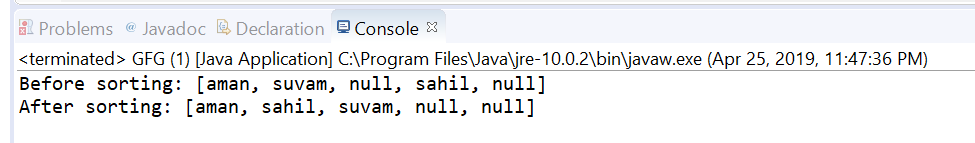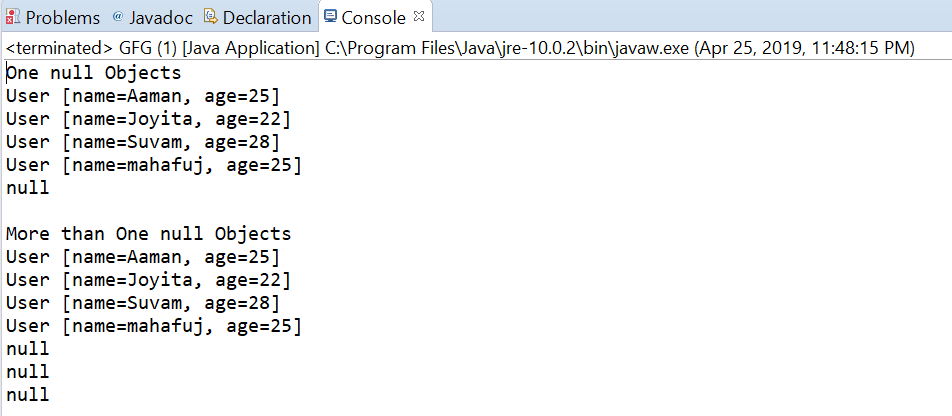Related Articles

# Comparator nullsLast() method in Java with examples

• Last Updated : 29 Apr, 2019

The nullsLast (java.util.Comparator) method returns comparator that is a null-friendly comparator and considers null values greater than non-null. The null first operates by the following logic:

1. The null element is considered to be greater than non-null.
2. When both elements are null, then they are considered equal.
3. When both elements are non-null, the specified Comparator determines the order.
4. If specified comparator is null, then the returned comparator considers all non-null elements equal.
5. The returned comparator is serializable if the specified comparator is serializable.

Syntax:

```static <T> Comparator<T> nullsLast (Comparator<T> comparator)
```

Parameters: This method accepts a single parameter comparator which is a Comparator for comparing non-null values

Return value: This method returns a comparator that considers null to be greater than non-null and compares non-null objects with the supplied Comparator.

Below programs illustrate nullsLast(java.util.Comparator) method:
Program 1:

 `// Java program to demonstrate``// Comparator.nullsLast (java.util.Comparator)  method`` ` `import` `java.util.Arrays;``import` `java.util.Comparator;`` ` `public` `class` `GFG {`` ` `    ``public` `static` `void` `main(String[] args)``    ``{`` ` `        ``// create a collection of an array of names``        ``// also contains nulls``        ``String[] strings = { ``"aman"``, ``"suvam"``, ``null``,``                             ``"sahil"``, ``null` `};`` ` `        ``// print the array``        ``System.out.println(``"Before sorting: "``                           ``+ Arrays.toString(strings));`` ` `        ``// apply nullsLast  method``        ``// and sort the array``        ``Arrays.sort(strings,``                    ``Comparator.nullsLast(``                        ``Comparator.naturalOrder()));`` ` `        ``// print the array``        ``System.out.println(``"After sorting: "``                           ``+ Arrays.toString(strings));``    ``}``}`

The output printed on console of IDE is shown below.
Output:Program 2:

 `// Java program to demonstrate``// Comparator.nullsLast (java.util.Comparator)  method`` ` `import` `java.util.Arrays;``import` `java.util.Collections;``import` `java.util.Comparator;``import` `java.util.List;``public` `class` `GFG {``    ``public` `static` `void` `main(String[] args)``    ``{`` ` `        ``// create some user objects``        ``User u1 = ``new` `User(``"Aaman"``, ``25``);``        ``User u2 = ``new` `User(``"Joyita"``, ``22``);``        ``User u3 = ``new` `User(``"Suvam"``, ``28``);``        ``User u4 = ``new` `User(``"mahafuj"``, ``25``);`` ` `        ``System.out.println(``"One null Objects"``);``        ``List list``            ``= Arrays.asList(u1, u2, u3, ``null``, u4);``        ``Collections.sort(list,``                         ``Comparator.nullsLast(``                             ``Comparator.comparing(``                                 ``User::getName)));``        ``list.forEach(user -> System.out.println(user));`` ` `        ``System.out.println(``"\nMore than One null Objects"``);``        ``list = Arrays.asList(u1, u4, ``null``, u2, u3, ``null``, ``null``);``        ``Collections.sort(list,``                         ``Comparator.nullsLast(``                             ``Comparator.comparing(``                                 ``User::getName)));``        ``list.forEach(user -> System.out.println(user));``    ``}``}``class` `User ``implements` `Comparable {``    ``public` `String name;``    ``public` `int` `age;`` ` `    ``public` `User(String name, ``int` `age)``    ``{``        ``this``.name = name;``        ``this``.age = age;``    ``}`` ` `    ``public` `int` `compareTo(User u1)``    ``{``        ``return` `name.compareTo(u1.name);``    ``}`` ` `    ``public` `String getName()``    ``{``        ``return` `name;``    ``}`` ` `    ``public` `void` `setName(String name)``    ``{``        ``this``.name = name;``    ``}`` ` `    ``public` `int` `getAge()``    ``{``        ``return` `age;``    ``}`` ` `    ``public` `void` `setAge(``int` `age)``    ``{``        ``this``.age = age;``    ``}`` ` `    ``@Override``    ``public` `String toString()``    ``{``        ``return` `"User [name="` `+ name``            ``+ ``", age="` `+ age + ``"]"``;``    ``}``}`

The output printed on console is shown below.
Output:Attention reader! Don’t stop learning now. Get hold of all the important Java Foundation and Collections concepts with the Fundamentals of Java and Java Collections Course at a student-friendly price and become industry ready. To complete your preparation from learning a language to DS Algo and many more,  please refer Complete Interview Preparation Course.

My Personal Notes arrow_drop_up